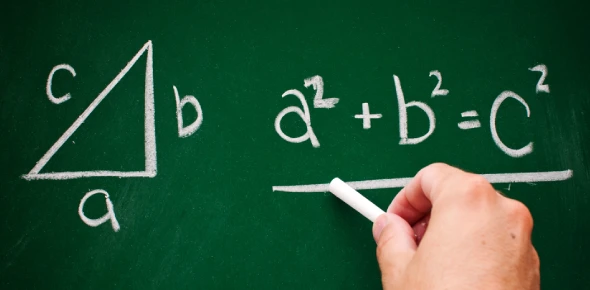# Square(S) No 1 (2 To 15) Test Your Self

Approved & Edited by ProProfs Editorial Team
At ProProfs Quizzes, our dedicated in-house team of experts takes pride in their work. With a sharp eye for detail, they meticulously review each quiz. This ensures that every quiz, taken by over 100 million users, meets our standards of accuracy, clarity, and engagement.
| Written by Hetal
H
Hetal
Community Contributor
Quizzes Created: 1 | Total Attempts: 64
Questions: 14 | Attempts: 64SettingsIF you are taking any entrance test for college level here is the few basic stuff you should know by your heart. Well this is from middle school however still you should remember.

• 1.

### 2 x 2 =

• A.

3

• B.

4

• C.

5

• D.

2 x 2

• E.

1.999999

B. 4
Explanation
The correct answer is 4 because when you multiply 2 by 2, you get the product of 4.

Rate this question:

• 2.

### 3 x 3 =

• A.

7

• B.

8

• C.

8.99999999

• D.

9

• E.

9.00001

D. 9
Explanation
The given question asks for the product of 3 multiplied by 3. The correct answer is 9, which is obtained by multiplying the two numbers together.

Rate this question:

• 3.

### 4 x4 =

• A.

16

• B.

16.001

• C.

17

• D.

-16

• E.

15.0001

A. 16
Explanation
The correct answer is 16 because when you multiply 4 by 4, you get the product of 16.

Rate this question:

• 4.

### 5 x 5 =

• A.

-24

• B.

24

• C.

-25

• D.

25

• E.

25.001

D. 25
Explanation
The question asks for the product of 5 multiplied by 5. The correct answer is 25 because when you multiply 5 by 5, you get 25.

Rate this question:

• 5.

### 6 x 6=

• A.

36.3636

• B.

36

• C.

-35.00

• D.

35

• E.

34.999999

B. 36
Explanation
The correct answer is 36 because when you multiply 6 by 6, you get 36.

Rate this question:

• 6.

### 7 x 7=

• A.

36

• B.

59

• C.

49

• D.

48

• E.

47

C. 49
Explanation
The correct answer is 49 because when you multiply 7 by itself, you get 49.

Rate this question:

• 7.

### 8 x 8 =

• A.

46

• B.

64

• C.

-46

• D.

0.46

• E.

46.001

B. 64
Explanation
The correct answer is 64 because when you multiply 8 by 8, you get the product of 64.

Rate this question:

• 8.

### 9 x 9 =

• A.

71

• B.

79

• C.

81

• D.

-82

• E.

82.001

C. 81
Explanation
The correct answer is 81 because 9 multiplied by 9 equals 81.

Rate this question:

• 9.

### 10 x 10 =

• A.

10000

• B.

0.10000

• C.

-100

• D.

100

• E.

-0.100

D. 100
Explanation
The correct answer is 100 because when you multiply 10 by 10, you get 100.

Rate this question:

• 10.

### 11 x 11 =

• A.

121

• B.

111

• C.

1212

• D.

211

• E.

-111

A. 121
Explanation
The correct answer is 121. This is because when you multiply 11 by itself, you get 121.

Rate this question:

• 11.

### 12 x 12 =

• A.

143

• B.

142

• C.

441

• D.

134

• E.

144

E. 144
Explanation
The correct answer is 144 because when you multiply 12 by 12, you get the product of 144.

Rate this question:

• 12.

### 13 x 13 =

• A.

149

• B.

159

• C.

129

• D.

169

• E.

-159

D. 169
Explanation
The correct answer is 169 because when you multiply 13 by 13, you get the product of 169.

Rate this question:

• 13.

### 14 x 14 =

• A.

169

• B.

176

• C.

156

• D.

196

• E.

206

D. 196
Explanation
The correct answer is 196. This is because when you multiply 14 by 14, you get 196.

Rate this question:

• 14.

### 15 x 15 =

• A.

252

• B.

125

• C.

124

• D.

123

• E.

225Back to top